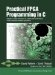# Section 13.4. Creating a Streaming Version

### 13.4. Creating a Streaming Version

At this point we have created a fixed-point version of the algorithm that might be appropriate for compiling to an embedded processor. If we want to move this algorithm to an FPGA, we first need to do some additional work to allow the configuration data to be transferred from the test routine (an equivalent of the existing main function) to the image generator and to allow the resulting pixel data to be transferred back out to the test routine. To do this, we will make some minor changes to the fix_mandelbrot routine and more substantial changes to the test routine by introducing a producer/consumer module, as demonstrated in previous chapters.

#### The Impulse C Process

The modified Mandelbrot image generator function is shown in Figure 13-4. Notice that this version of the generator (which is now an Impulse C process) does not include the file I/O operations. These operations will instead be performed on the software side of the application.

Examining this process in more detail, you see the following:

• The process run function declaration, including the two streams config_stream and pixel_stream.

• Declarations for all local variables in the form of unsigned 32-bit integers.

##### Figure 13-3. Mandelbrot generation, fixed-point version.
` void fix_mandelbrot(uint32 xmax, uint32 xmin, uint32 ymax, uint32 ymin,                     uint32 dx, uint32 dy, uint32 hdx, uint32 hdy) {   uint32 max_iterations = MAX_ITERATIONS;   uint32 c_imag,c_real,two;   int j, i, t, R, G, B;   uint32 k, result, tmp, z_real, z_imag;   // BMP file opened here, etc...   two = 2*(1<<FRACBITS);    // FRACBITS defined as 24   // Calculate points   c_imag = ymax;   for (j = 0; j < YSIZE; j++) {      c_real = xmin;      for (i = 0; i < XSIZE; i++) {         z_real = z_imag = 0;         // Calculate z0, z1, .... until divergence or max_iterations         k = 0;         do {           tmp = FXMUL(z_real,z_real);           tmp = FXSUB(tmp,FXMUL(z_imag,z_imag));           tmp = FXADD(tmp,c_real);           z_imag = FXMUL(two,FXMUL(z_real,z_imag));           z_imag = FXADD(z_imag,c_imag);           z_real = tmp;           tmp = FXMUL(z_real,z_real);           result = FXADD(tmp,FXMUL(z_imag,z_imag));           k++;         } while (result < (4*(1<<FRACBITS)) && k < max_iterations);         // Map points to gray scale: change to suit your preferences         B = G = R = 0;         if (k != MAX_ITERATIONS) {             R = G = G = k > 255 ? 255 : k;         }         putc(B, outfile); putc(G, outfile); putc(R, outfile);         c_real = FXADD(c_real,dx);       }       c_imag = FXSUB(c_imag,dy);   }   fclose(outfile); } `

##### Figure 13-4. Mandelbrot generation, Impulse C version.
` void mandelbrot(co_stream config_stream, co_stream pixel_stream) {   co_uint32 xmax,xmin,ymax,ymin,dx,dy,hdx,hdy;   co_uint32 B,G,R,BGR;   co_uint32 i,j,k,t;   co_uint32 c_imag,c_real,two,four;   co_uint32 result,tmp;   co_uint32 z_real,z_imag;   two = FXCONST(2);   four = FXCONST(4);   co_stream_open(config_stream, O_RDONLY, UINT_TYPE(32));   // Read in parameters   while (co_stream_read(config_stream, &xmax, sizeof(co_uint32)) ==                                                      co_err_none) {     co_stream_read(config_stream,&xmin,sizeof(co_uint32));     co_stream_read(config_stream,&ymax,sizeof(co_uint32));     co_stream_read(config_stream,&ymin,sizeof(co_uint32));     co_stream_read(config_stream,&dx,sizeof(co_uint32));     co_stream_read(config_stream,&dy,sizeof(co_uint32));     co_stream_read(config_stream,&hdx,sizeof(co_uint32));     co_stream_read(config_stream,&hdy,sizeof(co_uint32));     IF_SIM(printf("x: %f - %f\n",FX2REAL(xmin),FX2REAL(xmax));)     IF_SIM(printf("y: %f - %f\n",FX2REAL(ymin),FX2REAL(ymax));)     // Loop over region     co_stream_open(pixel_stream, O_WRONLY, UINT_TYPE(24));     c_imag = ymax;     for (j=0; j<YSIZE; j++) {        c_real = xmin;        for (i=0; i<XSIZE; i++) {           z_real = z_imag = 0;           // Calculate point           k = 0;           do {             tmp = FXMUL(z_real,z_real);             tmp = FXSUB(tmp,FXMUL(z_imag,z_imag));             tmp = FXADD(tmp,c_real);             z_imag = FXMUL(two,FXMUL(z_real,z_imag));             z_imag = FXADD(z_imag,c_imag);             z_real = tmp;             tmp = FXMUL(z_real,z_real);             result = FXADD(tmp,FXMUL(z_imag,z_imag));             k++;          } while ((result < four) && (k < MAX_ITERATIONS));          // Map points to gray scale: change to suit your preferences          B = G = R = 0;          if (k != MAX_ITERATIONS) {              R = G = G = k > 255 ? 255 : k;          }          BGR = ((B<<16) & BLUEMASK) |                ((G<<8) & GREENMASK) |                 (R & REDMASK);          co_stream_write(pixel_stream,&BGR,sizeof(co_uint32));          c_real = FXADD(c_real,dx);        }        c_imag = FXSUB(c_imag,dy);       }       co_stream_close(pixel_stream);   }   co_stream_close(config_stream); } `

• Assignments (to variables named two and four) of constant fixed-point representations for the values 2 and 4, respectively. These constants are defined using the FXCONST macro, which is declared elsewhere (in mand.h) as FXCONST32(a,FRACBITS). Recall that FRACBITS has been defined for this application as 24, indicating the number of bits associated with the fractional part of a number.

• A call to co_stream_open, which opens the configuration stream. The configuration stream accepts seven input values that collectively define the region of the X-Y plane to be processed.

• A co_stream_open function call corresponding to the pixel_stream output stream. Notice that this stream has a width of 24 bits. This corresponds to the three 8-bit color values that will be calculated for each pixel in the generated image.

• A nested loop of size (YSIZE times XSIZE) that processes the image line-by-line and pixel-by-pixel to produce the desired pattern.

• An inner code loop (the do loop) performs the iterative calculations for each pixel using fixed-point versions of the add, subtract, multiply, and divide operations.

• After this do loop, color values are assigned based on the number of iterations required for that pixel.

• At the completion of the nested loops (the processing of the entire image), the output stream is closed, and a new set of configuration data is read, continuing the operation repeatedly until it has been detected that the configuration stream is closed.

The result of this process is a stream of pixel values that begin to appear on the process output stream (pixel_stream) after the seven input values have been read into the process input stream (config_stream).

We now have a process that can be compiled to hardware; if we also provide a software test bench, we have a complete application that may be simulated within a standard C environment or tested in-system using an embedded processor, as described in previous chapters. Before doing so, however, let's look at how we might improve this process by introducing more parallelism.Practical FPGA Programming in C
ISBN: 0131543180
EAN: 2147483647
Year: 2005
Pages: 208

Similar book on Amazon